Dose Response Modelling in R

This tutorial is designed to give a basic introduction to applying dose-response models to continuous data in R using a combination of the tidyverse and drc packages. I’ll put up a visualising dose-response models tutorial a bit later!

It’s basically a more-anotated repeat of an example given by Dr Christian Ritz in his PLOS One article, who developed the drc package… with some additional tidbits that I’ve found helpful over the years. It’s not meant to be exhaustive and should not replace the excellent package documentation and accompanying paper.

## Setting up

Here are the packages we need:

library(tidyverse)
library(drc)

Importing data is it’s own struggle if you’re new to R. Using R Studio (an interface to R) and R Projects will make your life easier. For this example, I’ll be using a dataset that is already in the drc package called “ryegrass”.

toxdata<- ryegrass
str(ryegrass)
## 'data.frame':    24 obs. of  2 variables:
##  $rootl: num 7.58 8 8.33 7.25 7.38 ... ##$ conc : num  0 0 0 0 0 0 0.94 0.94 0.94 1.88 ...

As we can see, the data contains 24 observations of two variables, a response which reports root length “rootl” and a herbicide dose “conc”. The drc package makes generalised nonlinear modelling super simple:

model<- drm(rootl~conc, data=ryegrass, fct=LL.4(names = c("Slope", "Lower Limit", "Upper Limit", "ED50")))
#you don't need the 'names = ' argument but it's useful to label the b, c, d, and e parameters until you're familiar with
plot(model, type="all")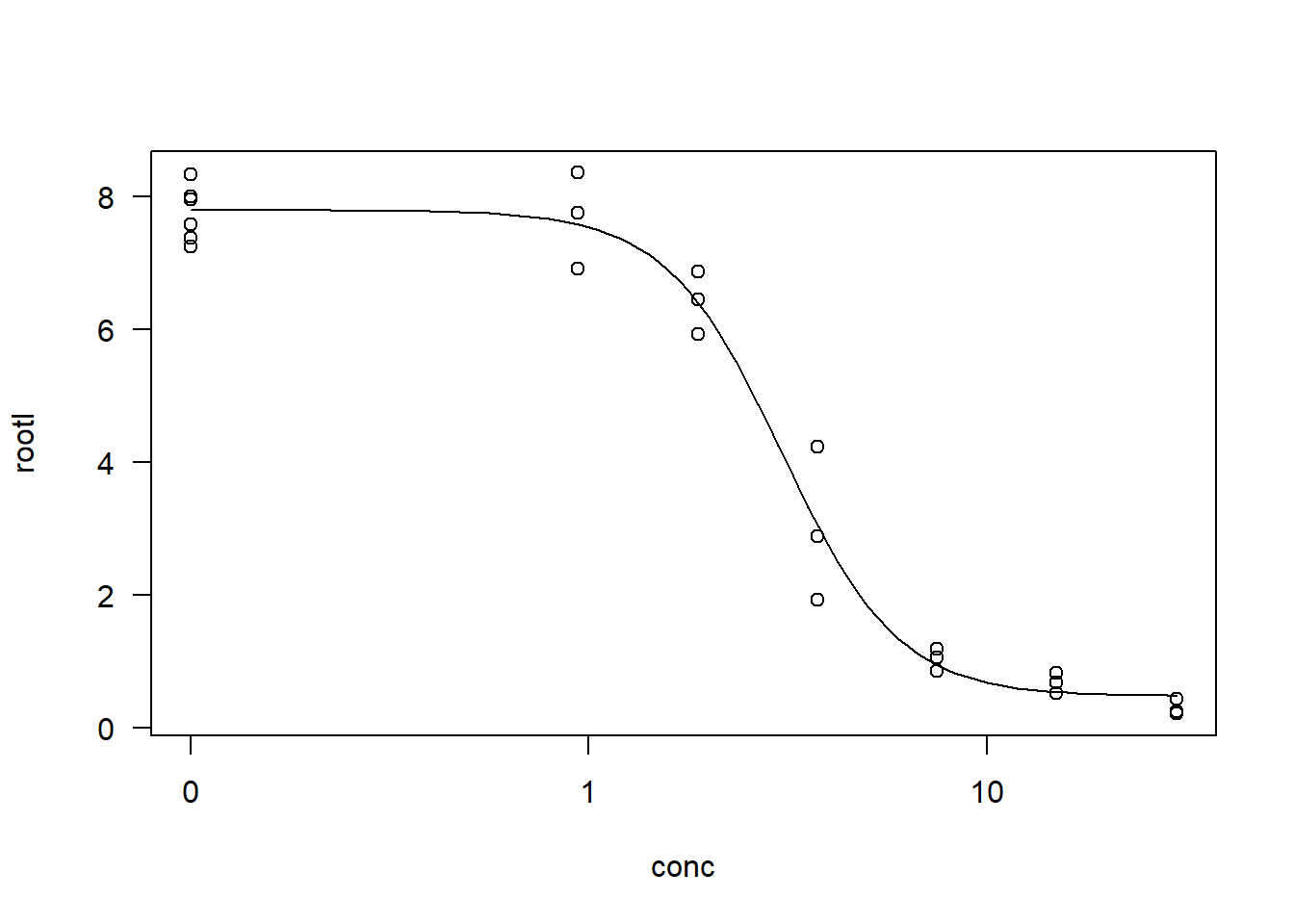#the "type = 'all'" argument displays all data points on the plot..the default is to hide some. I'm really not sure why. 

Here we’ve fitted a 4-parameter log-logistic model to the data. We can get a summary of the model parameters using the summary function and calculate the EC10, EC20, EC50 etc with the ED function.

summary(model)
##
## Model fitted: Log-logistic (ED50 as parameter) (4 parms)
##
## Parameter estimates:
##
##                         Estimate Std. Error t-value   p-value
## Slope:(Intercept)        2.98222    0.46506  6.4125 2.960e-06 ***
## Lower Limit:(Intercept)  0.48141    0.21219  2.2688   0.03451 *
## Upper Limit:(Intercept)  7.79296    0.18857 41.3272 < 2.2e-16 ***
## ED50:(Intercept)         3.05795    0.18573 16.4644 4.268e-13 ***
## ---
## Signif. codes:  0 '***' 0.001 '**' 0.01 '*' 0.05 '.' 0.1 ' ' 1
##
## Residual standard error:
##
##  0.5196256 (20 degrees of freedom)
#interval = "delta" gives you asymptotically-based confidence intervals at a default 95% level.
ED(model, c(10,20,50), interval="delta")
##
## Estimated effective doses
##
##        Estimate Std. Error   Lower   Upper
## e:1:10  1.46371    0.18677 1.07411 1.85330
## e:1:20  1.92109    0.17774 1.55032 2.29186
## e:1:50  3.05795    0.18573 2.67053 3.44538

It look greats, it fits, it works. But how often do you have great data? My data are rarely great. Differemt models provide different fits which could improve the accuracy of your estimates. The drc package has a number of in-built models ready to use that will cover most of your modelling needs.

## Crash course in model selection

The key models I use for my chronic and acute toxicity data are:

• Log logistic
• Weibull type 1
• Asymmetrical, its first shoulder (at the EC05-EC10) starts later than a log-logistic
• Weibull type 2
• Asymmetrical, its first shoulder (at the EC05-EC10) starts earlier than a log-logistic model

Each of these have the same parameters

• b, Slope (between the EC10 and EC90)
• c, Upper limit
• d, Lower limit
• e, Mid point (aka ED50 but more on that later)

Each can account for different data differently, but follow the same sigmoidal shape where there’s no response, a response, and no more response. There’s no mechanistic basis for these models in ecotoxicology. They’re used because they fit so work with whatever fits best.

### How many parameters is best?

In short, the fewer parameters the better - this is the law of parsimony in modelling. You could have 0 residual error in a model that connects the dots of your data, but that wouldn’t be useful for describing the overall population trends.

The drm package gives you the option of models with different numbers of paramters. Log logistic models come in 2, 3, 4, or 5 parameter versions: LL.2(), LL.3(), LL.4(), or LL.5(), while Weibull type 1 and 2 models come in 3 or 4 parameter versions: W1.3()and W1.4() or W2.3() and W2.4(). For the most part, they’re based on the 4 parameter model described above. The 3 parameter versions just fix the lower limit to 0 (which is useful if your response can’t be negative like length or growth), and the 2 parameter version fixes both the lower limit to 0 and the upper limit to 1 (which is mostly used for binary data, like survival).

In my work, I normally normalise the growth rates of algae to an experimental control growth rate to account for inter-test variability. This means I have a reason to fix the upper limit to 100, because by normalising the data I have explicitly defined it as starting at 100 at a dose of 0. To fix model paramters, you can use the argument “fixed”:

#start by converting all the responses into a percent of the control response
toxdata <- toxdata %>%
mutate(percent_response = rootl/(mean(toxdata$rootl[toxdata$conc==0]))*100)
#check that we now have a response out of 100
head(toxdata)
##      rootl conc percent_response
## 1 7.580000    0         97.81472
## 2 8.000000    0        103.23453
## 3 8.328571    0        107.47452
## 4 7.250000    0         93.55629
## 5 7.375000    0         95.16933
## 6 7.962500    0        102.75062
#when fixing model parameters, we use "NA" to indicate that it needs to be calculated
model_fixed<- drm(percent_response~conc, data=toxdata,
fct=LL.4(fixed=c(NA, 0, 100, NA),
names = c("Slope", "Lower Limit", "Upper Limit", "ED50")))

par(mfrow = c(1, 2))
plot(model_fixed, main="LL.4(fixed=c(NA, 0, 100, NA))")

#this is the exact same model as the following log-logistic 3 parameter model
model_fixed2<- model_fixed<- drm(percent_response~conc, data=toxdata,
fct=LL.3(fixed=c(NA, 100, NA),
names = c("Slope", "Upper Limit", "ED50")))

plot(model_fixed2, main="LL.3(fixed=c(NA, 100, NA))")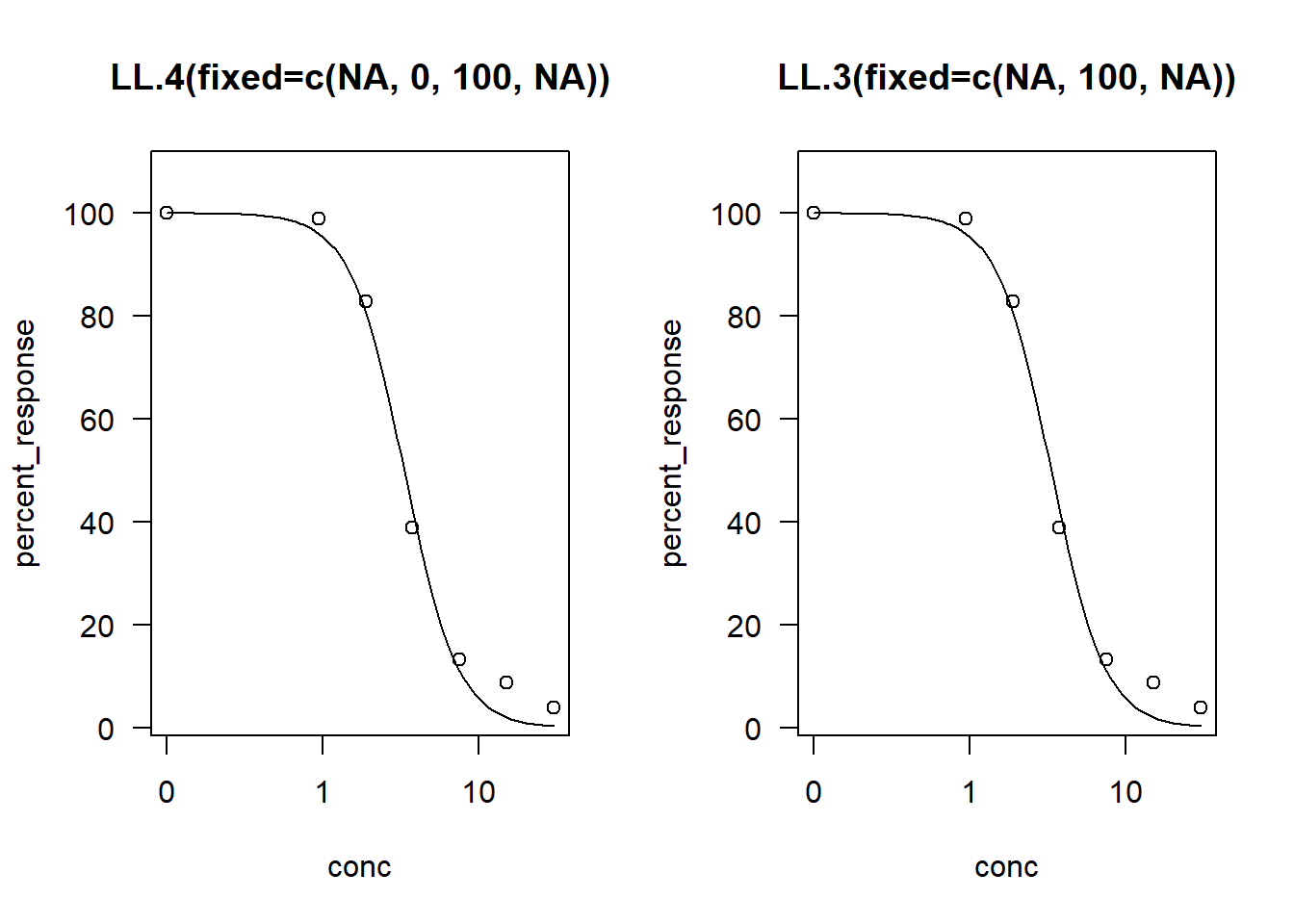They’re actually both 2-parameter models because only the slope and ED50 value are being fitted

### Choosing a model

The drc package is kind enouh to have a function made to compare models, ‘mselect’ which is a part of the drc package. The key inputs include a model (so run any one first), a list of models you want to compare the initial model to “fctList”, and whether you want to chuck in a linear regression “linreg” (as well as cubic and quadratic). Because we’re using percent response as our response variable, I will fix three parameter models to start at 100.

model.LL3<- drm(percent_response~conc, data=toxdata, fct=LL.3(fixed=c(NA, 100, NA), names = c("Slope", "Upper Limit", "ED50")))
mselect(model.LL3, fctList = list(W1.3(fixed=c(NA, 100, NA)),W1.4(), W2.3(fixed=c(NA, 100, NA)), W2.4(),  LL.4()),linreg=TRUE) 
##           logLik       IC Lack of fit   Res var
## W2.3   -78.16041 162.3208  0.88550040  43.05600
## W2.4   -77.29500 164.5900  0.94507135  44.06626
## LL.4   -77.53663 165.0733  0.86648304  44.96256
## LL.3   -80.13975 166.2795  0.44544802  50.77718
## W1.4   -78.84868 167.6974  0.45056762  50.15749
## W1.3   -83.87511 173.7502  0.06283767  69.31956
## Cubic  -86.91576 183.8315          NA  98.24110
## Quad   -96.49706 200.9941          NA 207.90396
## Lin   -111.85703 229.7141          NA 713.76468

The mselect function reports four measures of model fit. Here’s what the package documentation says

For Akaike’s information criterion and the residual standard error: the smaller the better and for lack-of-fit test (against a one-way ANOVA model): the larger (the p-value) the better. Note that the residual standard error is only available for continuous dose-response data. Log likelihood values cannot be used for comparison unless the models are nested.

For the most part, I care about the “IC” (which defaults to the Akaike’s Information Criterion, AIC). The rule of thumb is that the smaller the better and differences of more than 10 indicates “significantly” better model fits. Our results here suggest the Weibull 2.3 (W2.3()) provides the best fit, but is roughly as good as the W2.4, LL.4, LL.3, and W1.4 models (IC value <10 difference).

This is what they look like:

model.W23 <-  drm(percent_response~conc, data=toxdata, fct=W2.3(fixed=c(NA, 100, NA), names = c("Slope", "Upper Limit",  "ED50")))
model.W24 <-  drm(percent_response~conc, data=toxdata, fct=W2.4(names = c("Slope", "Lower Limit", "Upper Limit", "ED50")))
model.LL4 <-  drm(percent_response~conc, data=toxdata, fct=LL.4(names = c("Slope", "Lower Limit", "Upper Limit", "ED50")))
model.W14 <-  drm(percent_response~conc, data=toxdata, fct=W1.4(names = c("Slope", "Lower Limit", "Upper Limit", "ED50")))

plot(model.LL3, broken = TRUE, xlab="Concentration", ylab="Percent Response", type='all',lty=1, lwd=2)
plot(model.W14, add=TRUE,col="pink",lty=2, lwd=2)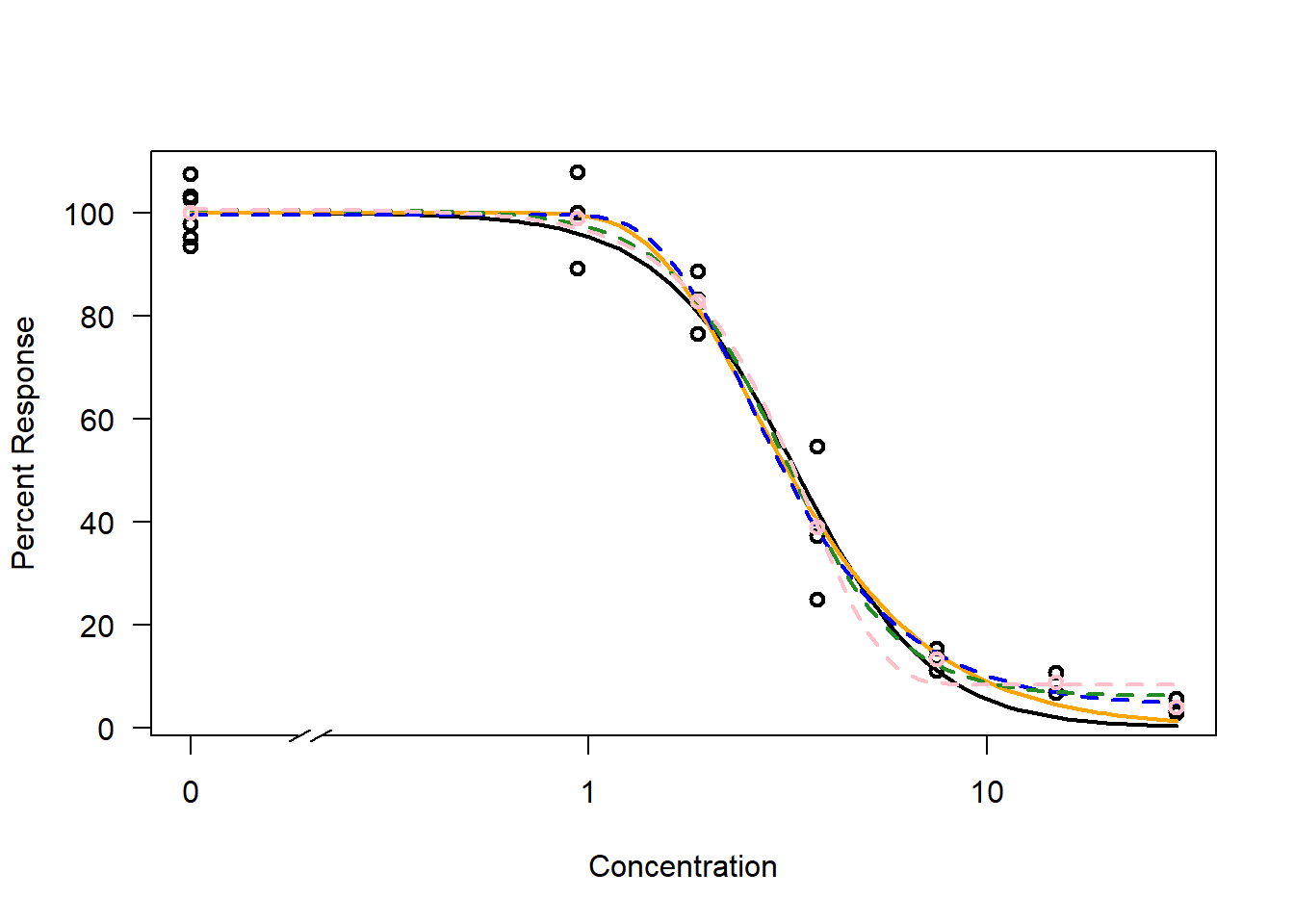In this plot, check out the:

• Lower asymptote - See how only the black and orange (solid line) models descend to 0? Thats the 3-parameter models fixing the lower limit to 0. Other models have decided the lower asymptote is around 10%. You have to make a decision whether that makes sense for you or not. It will impact how the the effect concentrations are caluclated.

• Upper asymptote - The control data was normalised to a mean of 100 and there isn’t much data between concentrations of 0 and 1, so all models are starting at around 100. This isn’t always the case. Data can be messy and the models will choose their own upper limit unless you fix that parameter to something that makes sense for your data.

If we go back to using root length as the response variable, we can see slight differences in where the models start and end. These differences can be large depending on the dataset so it’s important to have a think about what is appropriate for your work.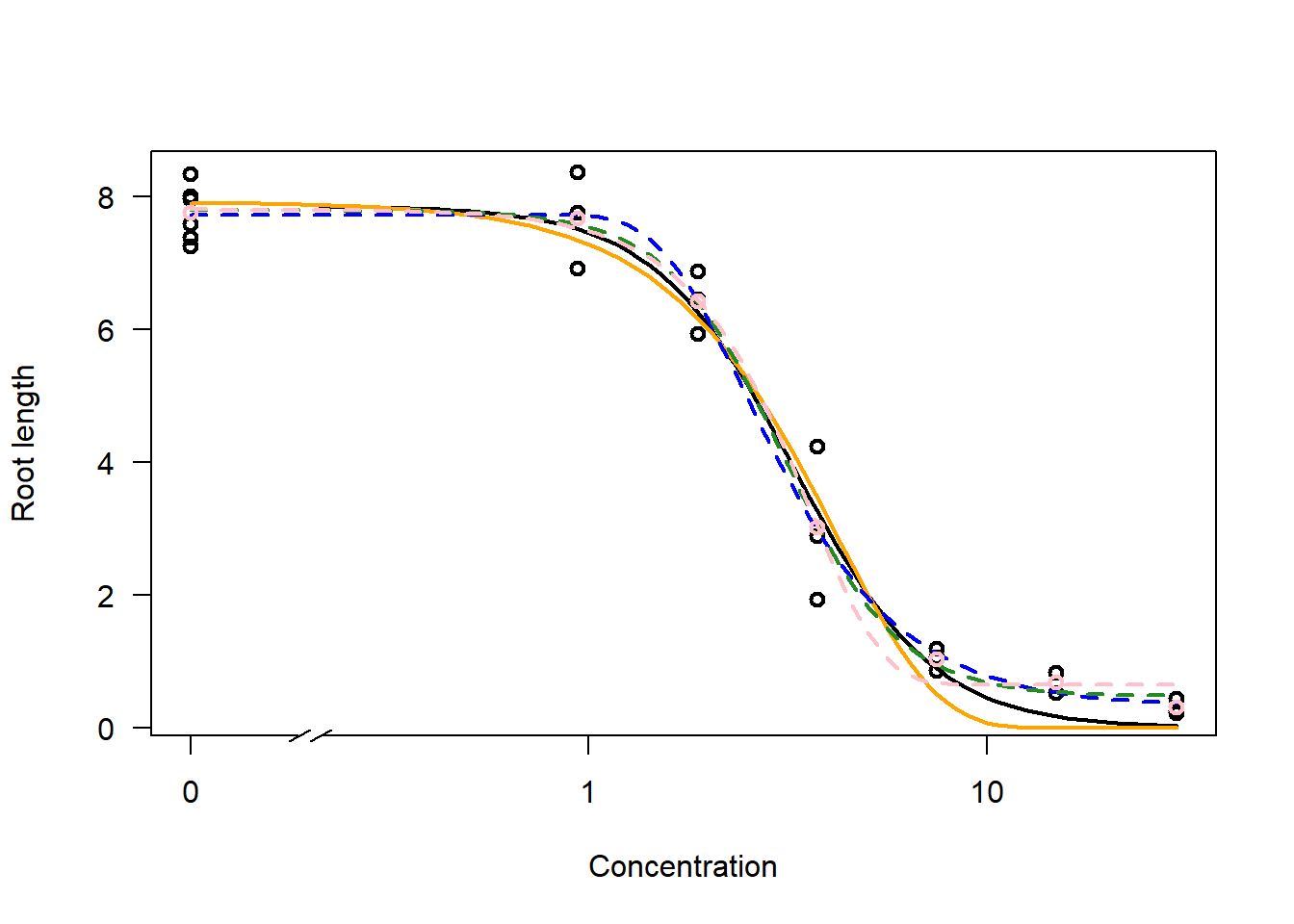## Calculating effective dose values

In ecotoxicological modelling we’re normally after an effective concentration, inhibition concentration,lethal dose, effective dose, or any other name. They all refer to the concentration required to cause a specific response. I.e. the ED10 is the concentration required to reduced the response by 10%.

For our data, it’s clear that the W2.3 model with an upper limit fixed at 100 (for percent response data): 1. makes sense for our data and experimental design 2. fits the data 3. is a parsimonious choice

To get our ED values we use a function in the drc package called “ED”. To use it, you tell it the model you want, give it a list of ED values you want to calculate, and tell it to calculate confidence intervals. Delta refers to the asymptotics-based method to calculate confidenc intervals. It works for all the models mentioned here!

ED(model.W23, c(10,50), interval="delta")
##
## Estimated effective doses
##
##        Estimate Std. Error   Lower   Upper
## e:1:10  1.57344    0.12742 1.30918 1.83771
## e:1:50  3.15766    0.15233 2.84175 3.47356

### Model averaging ED values

If you’re not sure which model to pick to calculate ED values… an option is to average all the models, weighted by how well they fit the data. Once again, the drc package has a function for model averaging effective doses “maED”.

The function’s arguments include a starting model, a list of models you want to average, the ED values you’re after, and a method to calculate the confidence intervals. This is different to the confidence interval above because we’re combining model predictions. There are two methods “kang” and “buckland” described in the package documentation. I normally go with Kang because I prefer the sound of confidence intervals based on “weighted means of confidence intervals for the individual fits” compared to “approximate variance formula under the assumption of perfectly correlated estimates”. I haven’t found either to be too different.

maED(model.W23,
list(W2.4(),LL.4(),LL.3(fixed=c(NA, 100, NA)), W1.4()),c(10, 50),
interval="kang")
##          ED10     ED50     Weight
## W2.3 1.573443 3.157658 0.56172139
## W2.4 1.628225 2.996920 0.18062322
## LL.4 1.463714 3.057958 0.14185221
## LL.3 1.392584 3.309842 0.07760714
## W1.4 1.405943 3.088952 0.03819604
##        Estimate    Lower    Upper
## e:1:10 1.547339 1.244449 1.850229
## e:1:50 3.123669 2.775180 3.472158

As you can see the resulting ED values are basically the same!

## Common pitfalls in dose-response modelling

### Curse of the data that doesnt go to 0

The ED function will calculate its ED values based on the relative distance between the upper and lower asymptotes. This can lead to some confusion. For example, if we have a model starting at 100 with a lower asymptote at 20, our calculated ED50 value will actually be the midpoint of 20 and 100, an ED60.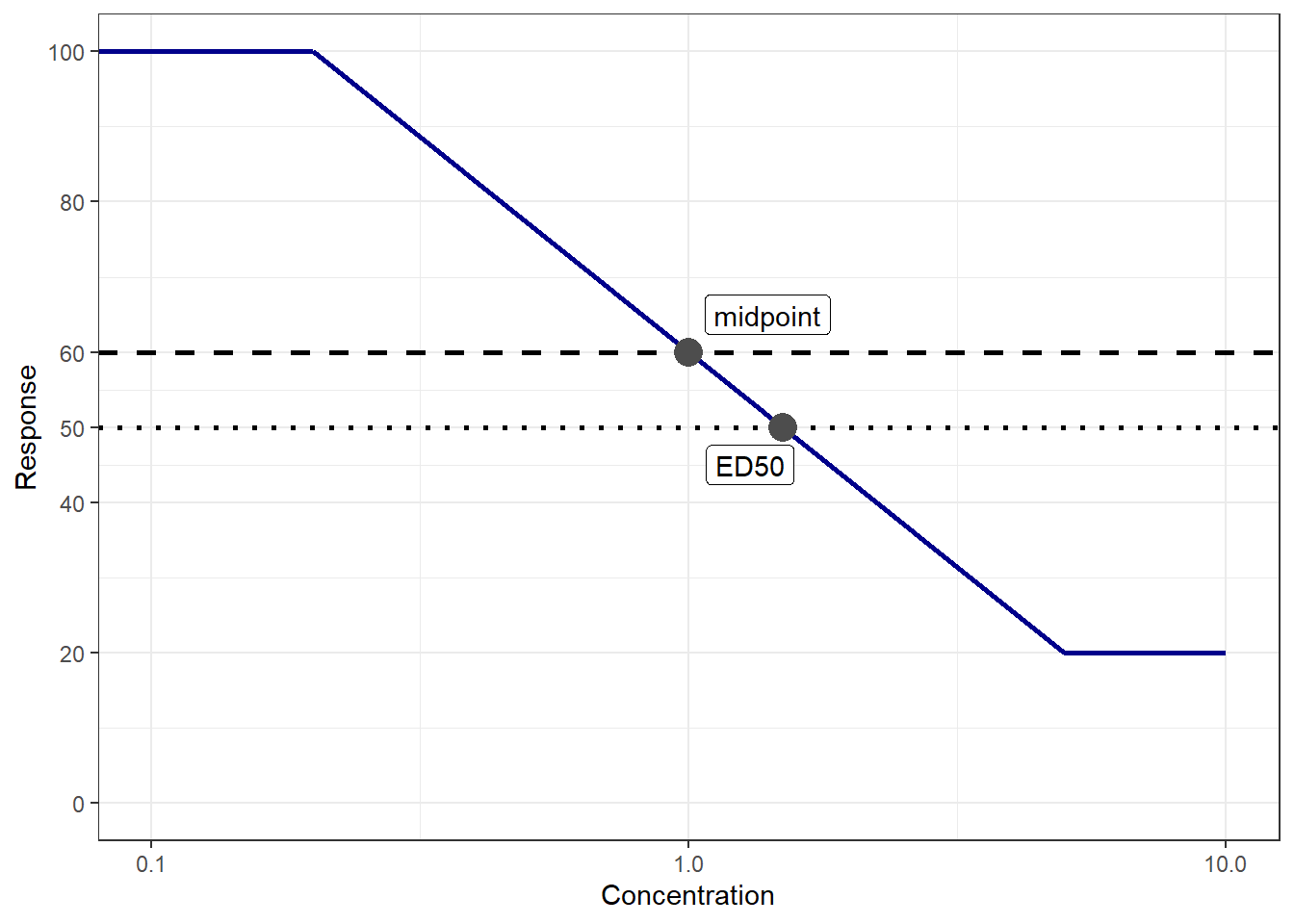Do you think anything is missing? Let me know in the comments below!